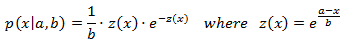class template
<random>

# std::extreme_value_distribution

`template <class RealType = double> class extreme_value_distribution;`
Extreme Value distribution
Random number distribution that produces floating-point values according to a Type I extreme value distribution, which is described by the following probability density function:This distribution produces random numbers where each value can be interpreted as the extreme (maximum or minimum) of a number of samples of a random variable.

The distribution parameters, a and b, are set on construction.

To produce a random value following this distribution, call its member function operator().

### Template parameters

RealType
A floating-point type. Aliased as member type result_type.
By default, this is double.

### Member types

The following aliases are member types of extreme_value_distribution:

member typedefinitionnotes
result_typeThe first template parameter (RealType)The type of the numbers generated (defaults to double)
param_typenot specifiedThe type returned by member param.

### Example

 ``12345678910111213141516171819202122232425262728293031`` ``````// extreme_value_distribution #include #include int main() { const int nrolls=10000; // number of experiments const int nstars=100; // maximum number of stars to distribute const int nintervals=20; // number of intervals const int first=-5; // first interval std::default_random_engine generator; std::extreme_value_distribution distribution(2.0,4.0); int p[nintervals]={}; for (int i=0; i=-5.0)&&(number<15.0)) ++p[int(number-first)]; } std::cout << "extreme_value_distribution (2.0,4.0):" << std::endl; for (int i=0; i

Possible output:
 ```extreme_value_distribution (2.0,4.0): -5..-4: -4..-3: * -3..-2: *** -2..-1: ***** -1.. 0: ******* 0.. 1: ******** 1.. 2: ******** 2.. 3: ********* 3.. 4: ******** 4.. 5: ******** 5.. 6: ****** 6.. 7: ***** 7.. 8: ***** 8.. 9: *** 9..10: *** 10..11: ** 11..12: ** 12..13: * 13..14: * 14..15: * ```